### Home > INT3 > Chapter 6 > Lesson 6.2.2 > Problem6-49

6-49.
1. Solve each equation for x. Homework Help ✎

1. 10x = 16

2. 10x = 41

3. 3x = 729

4. 10x = 101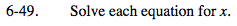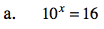Rewrite this equation in k = logbN form.

log1016 = x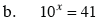See part (a).

$x \approx 1.613$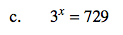What are some powers of 3? 34, 35, ...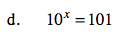See part (a).